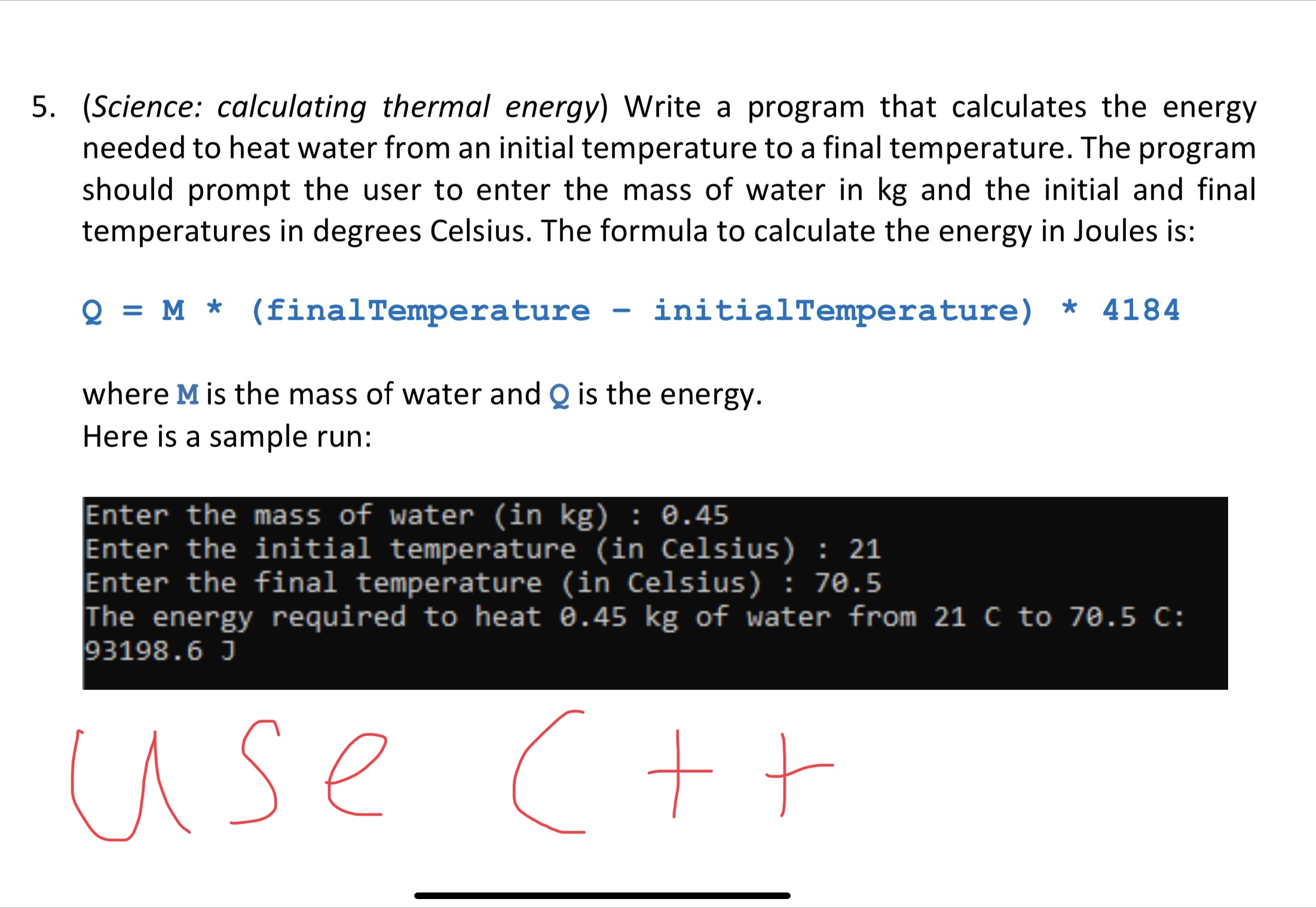Home / Expert Answers / Computer Science / science-calculating-thermal-energy-write-a-program-that-calculates-the-energy-needed-to-heat-wat-pa321

# (Solved): (Science: calculating thermal energy) Write a program that calculates the energy needed to heat wat ...(Science: calculating thermal energy) Write a program that calculates the energy needed to heat water from an initial temperature to a final temperature. The program should prompt the user to enter the mass of water in and the initial and final temperatures in degrees Celsius. The formula to calculate the energy in Joules is: where is the mass of water and is the energy. Here is a sample run: Enter the mass of water (in ) : 0.45 Enter the initial temperature (in Celsius) : 21 Enter the final temperature (in Celsius) : 70.5 The energy required to heat of water from to :

We have an Answer from Expert